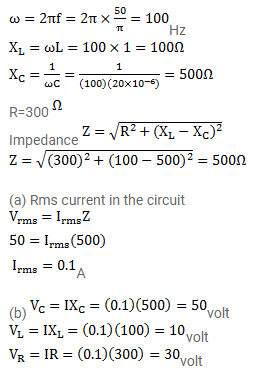# In a series LCR circuit with an AC source,

Question:

In a series LCR circuit with an $\mathrm{AC}$ source, $\mathrm{R}=300 \Omega, \mathrm{C}=20 \mu \mathrm{F}, \mathrm{L}=1.0$ henry, $\varepsilon_{\mathrm{rms}}=50 \mathrm{~V}$ and $\mathrm{v}=50 / \pi \mathrm{Hz}$. Find (a) the rms current in the circuit. (b) The rms potential differences across the capacitor, the resistor and the inductor. Note that the sum of the rms potential differences across the three elements is greater than the rms voltage of the source.

Solution: